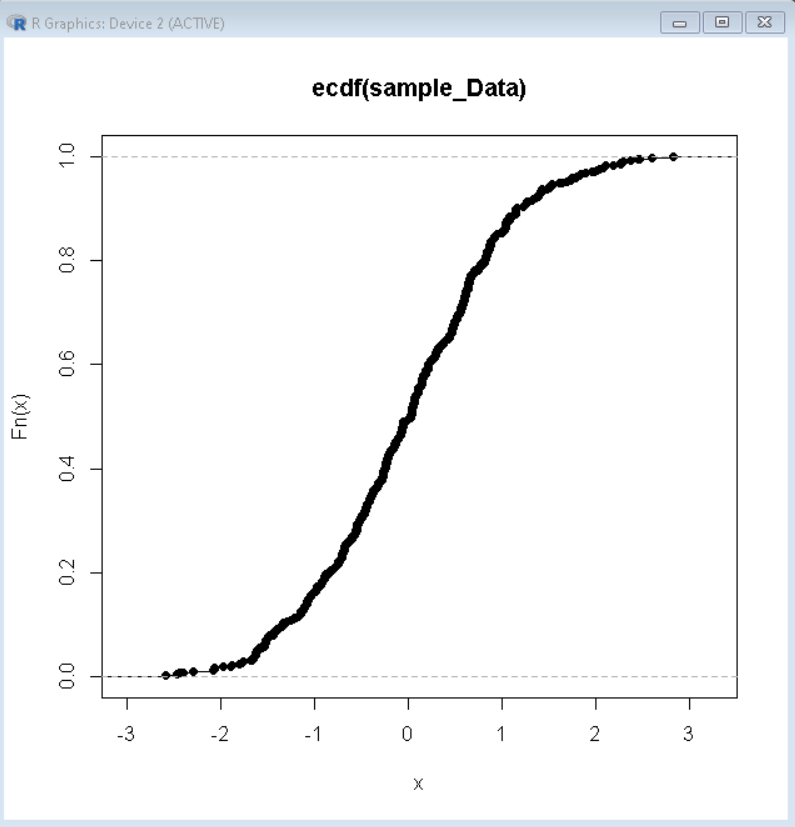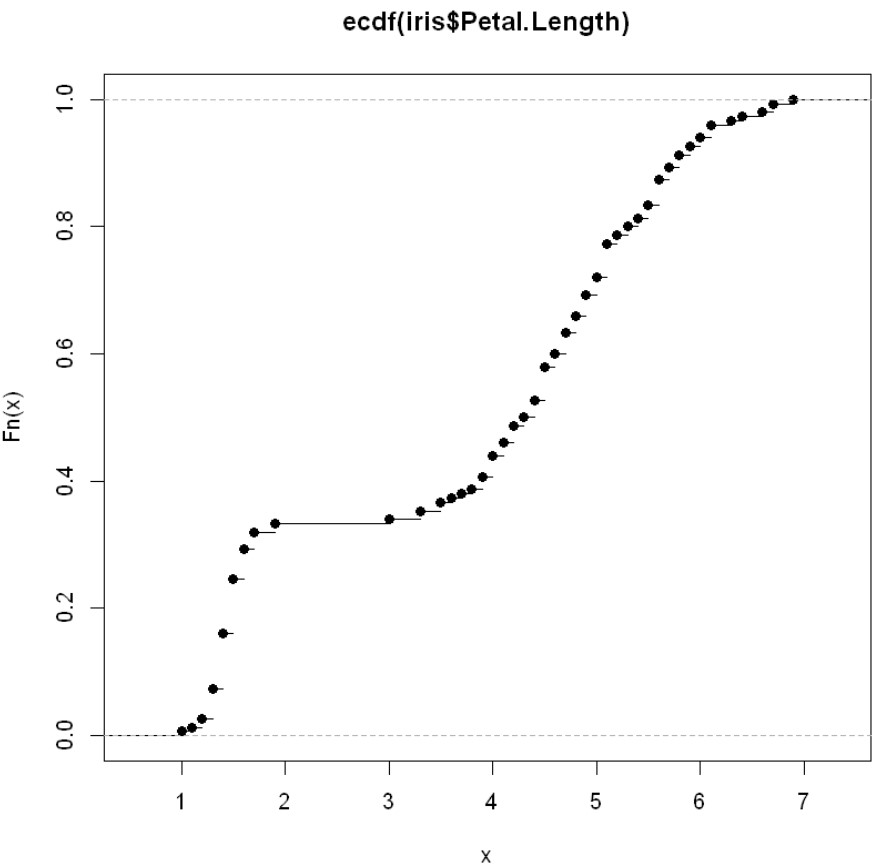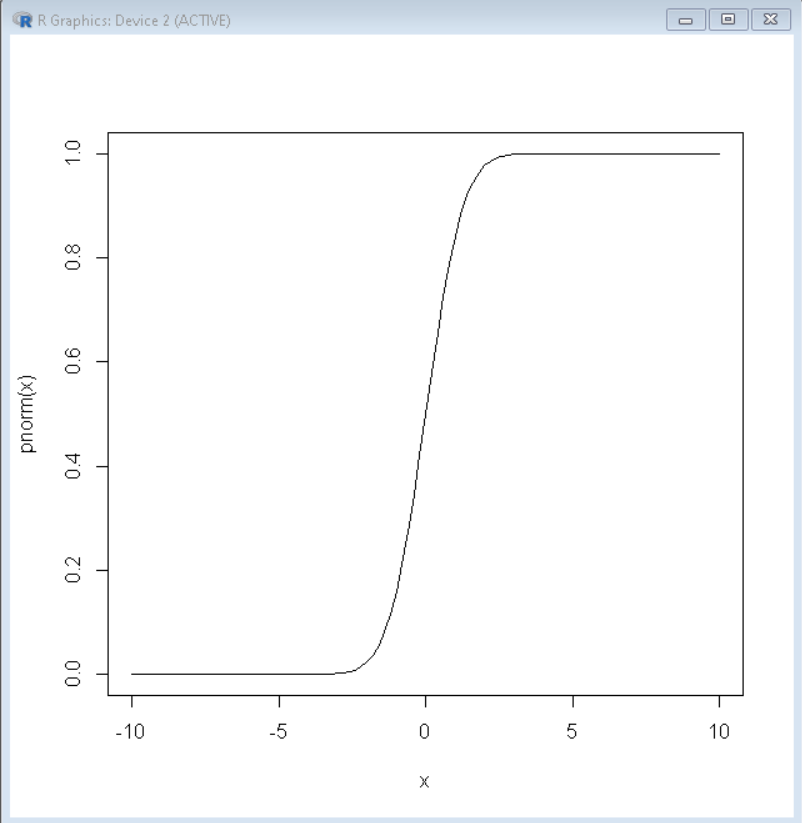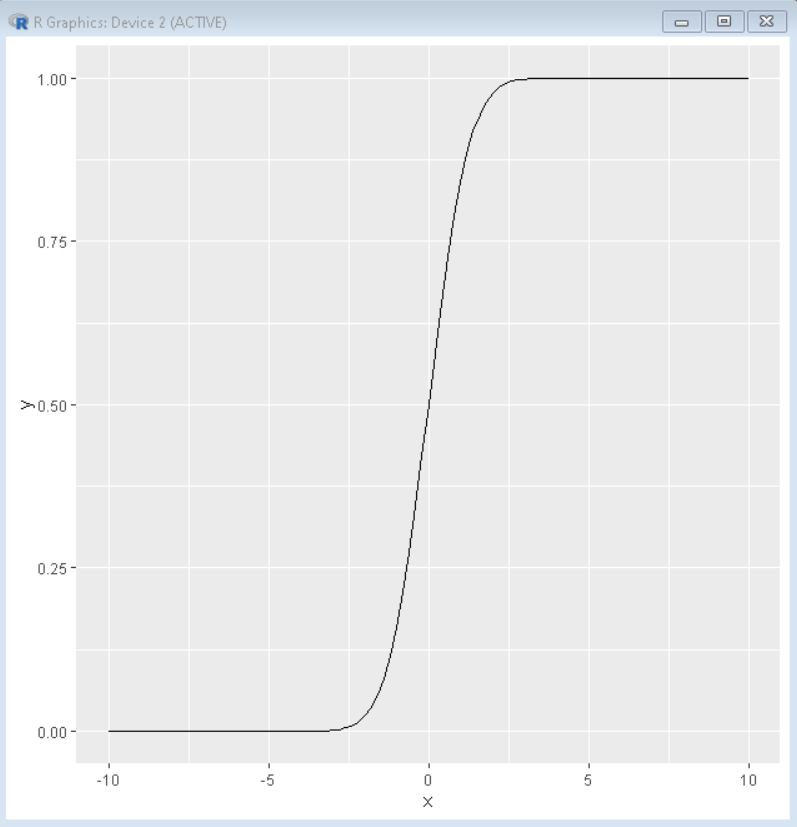# Plot Cumulative Distribution Function in R

• Last Updated : 30 Jan, 2022

In this article, we will discuss how to plot a cumulative distribution function (CDF) in the R programming Language.

The cumulative distribution function (CDF) of a random variable evaluated at x, is the probability that x will take a value less than or equal to x. To calculate the cumulative distribution function in the R Language, we use the ecdf() function. The ecdf() function in R Language is used to compute and plot the value of the Empirical Cumulative Distribution Function of a numeric vector. The ecdf() function takes the data vector as an argument and returns the CDF data.

Syntax: ecdf( data_vector )

Parameter:

• data_vector: determines the vector that contains data for CDF calculation.

## Plot cumulative distribution function in base R

To plot a CDF function in base R, we first calculate the CDF by using the ecdf() function. Then we use the plot() function to plot the CDF plot in the R Language. The plot function takes the result of the ecdf() function as an argument to plot the CDF plot.

Syntax: plot( CDF )

Parameter:

• CDF: determines the cumulative distribution function calculated using the ecdf() function.

### Example 1: Cumulative distribution function in base R

Here, is an example of a basic Cumulative Distribution Function Plot in the R Language.

## R

 `# create sample data``sample_Data = ``rnorm``(500)`` ` `# calculate CDF ``CDF <- ``ecdf``(sample_Data )`` ` `# draw the cdf plot``plot``( CDF )`

Output:## R

 `head``(iris)``plot``(``ecdf``(iris\$Petal.Length))`

Output:## Plot CDF of Known Distribution

To plot the cumulative distribution function of a standard distribution in a specific known range, we use the curve() function in the R Language. The curve() function draws a curve corresponding to a function over the interval. It takes an expression as an argument that in this case will be pnorm along with the limits from and to and returns a Normal CDF Plot.

Syntax: curve( expression, from, to )

Parameters:

• expression: determines the expression function for CDF calculation.
• from: determines the lower limit of data.
• to: determines the upper limit of data.

### Example:

Here, is an example of a normal CDF plot.

## R

 `# plot normal CDF plot``curve``(pnorm, from = -10, to = 10)`

Output:## Plot CDF of Known Distribution using ggplot2 Package

To draw the same plot in the ggplot2 package library, we use the stat_function() function. The stat_function takes the expression function as a fun argument and converts the curve according to that expression in a basic ggplot2 plot.

Syntax: plot + stat_function( fun )

Parameters:

• fun: determines the function for the shape of the plot.

### Example:

Here, is an example of a normal CDF plot using ggplot2.

## R

 `# load library ggplot2``library``(ggplot2)`` ` `# create sample dataframe for upper and lower limit``sample_limit<- ``data.frame``(x = ``c``(-10, 10))`` ` `# draw CDF Plot``ggplot``(sample_limit, ``aes``(x = x)) +``                  ``stat_function``(fun = pnorm)`

Output:My Personal Notes arrow_drop_up